# Maharashtra Board 12th Maths Solutions Chapter 7 Probability Distributions Ex 7.1

Balbharti 12th Maharashtra State Board Maths Solutions Book Pdf Chapter 7 Probability Distributions Ex 7.1 Questions and Answers.

## Maharashtra State Board 12th Maths Solutions Chapter 7 Probability Distributions Ex 7.1

Question 1.
Let X represent the difference between a number of heads and the number of tails when a coin is tossed 6 times. What are the possible values of X?
Solution:
When a coin is tossed 6 times, the number of heads can be 0, 1, 2, 3, 4, 5, 6.
The corresponding number of tails will be 6, 5, 4, 3, 2, 1, 0.
∴ X can take values 0 – 6, 1 – 5, 2 – 4, 3 – 3, 4 – 2, 5 – 1, 6 – 0
i.e. -6, -4, -2, 0, 2, 4, 6.
∴ X = {-6, -4, -2, 0, 2, 4, 6}.Question 2.
An urn contains 5 red and 2 black balls. Two balls are drawn at random. X denotes the number of black balls drawn. What are the possible values of X?
Solution:
The urn contains 5 red and 2 black balls.
If two balls are drawn from the urn, it contains either 0 or 1 or 2 black balls.
X can take values 0, 1, 2.
∴ X = {0, 1, 2}.

Question 3.
State which of the following are not the probability mass function of a random variable. Give reasons for your answer.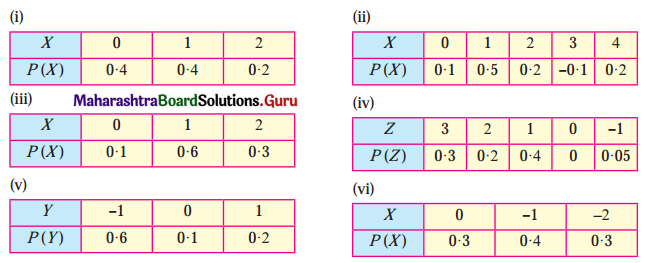Solution:
P.m.f. of random variable should satisfy the following conditions:
(a) 0 ≤ pi ≤ 1
(b) Σpi = 1.

(i)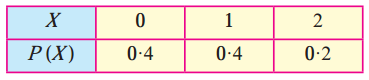(a) Here 0 ≤ pi ≤ 1
(b) Σpi = 0.4 + 0.4 + 0.2 = 1
Hence, P(X) can be regarded as p.m.f. of the random variable X.

(ii)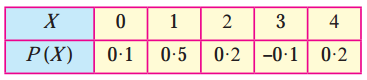P(X = 3) = -0.1, i.e. Pi < 0 which does not satisfy 0 ≤ Pi ≤ 1
Hence, P(X) cannot be regarded as p.m.f. of the random variable X.

(iii)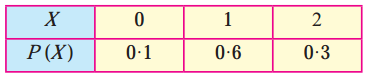(a) Here 0 ≤ pi ≤ 1
(b) ∑pi = 0.1 + 0.6 + 0.3 = 1
Hence, P(X) can be regarded as p.m.f. of the random variable X.

(iv)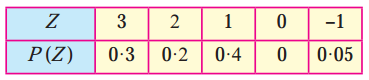Here ∑pi = 0.3 + 0.2 + 0.4 + 0 + 0.05 = 0.95 ≠ 1
Hence, P(Z) cannot be regarded as p.m.f. of the random variable Z.(v)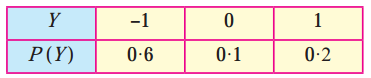Here ∑pi = 0.6 + 0.1 + 0.2 = 0.9 ≠ 1
Hence, P(Y) cannot be regarded as p.m.f. of the random variable Y.

(vi)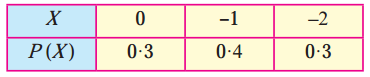(a) Here 0 ≤ pi ≤ 1
(b) ∑pi = 0.3 + 0.4 + 0.3 = 1
Hence, P(X) can be regarded as p.m.f. of the random variable X.

Question 4.
Find the probability distribution of
(i) number of heads in two tosses of a coin.
(ii) number of tails in the simultaneous tosses of three coins.
(iii) number of heads in four tosses of a coin.
Solution:
(i) For two tosses of a coin the sample space is {HH, HT, TH, TT}
Let X denote the number of heads in two tosses of a coin.
Then X can take values 0, 1, 2.
∴ P[X = 0] = P(0) = $$\frac{1}{4}$$
P[X = 1] = P(1) = $$\frac{2}{4}$$ = $$\frac{1}{2}$$
P[X = 2] = P(2) = $$\frac{1}{4}$$
∴ the required probability distribution is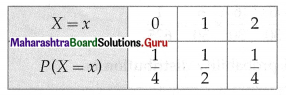(ii) When three coins are tossed simultaneously, then the sample space is
{HHH, HHT, HTH, THH, HTT, THT, TTH, TTT}
Let X denotes the number of tails.
Then X can take the value 0, 1, 2, 3.
∴ P[X = 0] = P(0) = $$\frac{1}{8}$$
P[X = 1] = P(1) = $$\frac{3}{8}$$
P[X = 2] = P(2) = $$\frac{3}{8}$$
P[X = 3] = P(3) = $$\frac{1}{8}$$
∴ the required probability distribution is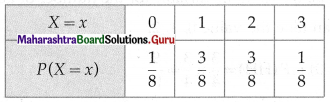(iii) When a fair coin is tossed 4 times, then the sample space is
S = {HHHH, HHHT, HHTH, HTHH, THHH, HHTT, HTHT, HTTH, THHT, THTH, TTHH, HTTT, THTT, TTHT, TTTH, TTTT}
∴ n(S) = 16
Let X denotes the number of heads.
Then X can take the value 0, 1, 2, 3, 4
When X = 0, then X = {TTTT}
∴ n(X) = 1
∴ P(X = 0) = $$\frac{n(X)}{n(S)}=\frac{1}{16}$$
When X = 1, then
X = {HTTT, THTT, TTHT, TTTH}
∴ n(X) = 4
∴ P(X = 1) = $$\frac{n(X)}{n(S)}=\frac{4}{16}=\frac{1}{4}$$
When X = 2, then
X = {HHTT, HTHT, HTTH, THHT, THTH, TTHH}
∴ n(X) = 6
∴ P(X = 2) = $$\frac{n(X)}{n(S)}=\frac{6}{16}=\frac{3}{8}$$
When X = 3, then
X = {HHHT, HHTH, HTHH, THHH}
∴ n(X) = 4
∴ P(X = 3) = $$\frac{n(X)}{n(S)}=\frac{4}{16}=\frac{1}{4}$$
When X = 4, then X = {HHHH}
∴ n(X) = 1
∴ P(X = 4) = $$\frac{n(X)}{n(S)}=\frac{1}{16}$$
∴ the probability distribution of X is as follows: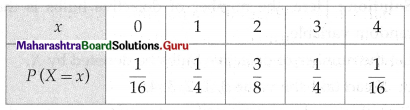Question 5.
Find the probability distribution of a number of successes in two tosses of a die, where success is defined as a number greater than 4 appearing on at least one die.
Solution:
When a die is tossed twice, the sample space s has 6 × 6 = 36 sample points.
∴ n(S) = 36
The trial will be a success if the number on at least one die is 5 or 6.
Let X denote the number of dice on which 5 or 6 appears.
Then X can take values 0, 1, 2.
When X = 0 i.e., 5 or 6 do not appear on any of the dice, then
X = {(1, 1), (1, 2), (1, 3), (1, 4), (2, 1), (2, 2), (2, 3), (2, 4), (3, 1), (3, 2), (3, 3), (3, 4), (4, 1), (4, 2), (4, 3), (4, 4)}
∴ n(X) = 16.
∴ P(X = 0) = $$\frac{n(X)}{n(S)}=\frac{16}{36}=\frac{4}{9}$$
When X = 1, i.e. 5 or 6 appear on exactly one of the dice, then
X = {(1, 5), (1, 6), (2, 5), (2, 6), (3, 5), (3, 6), (4, 5), (4, 6), (5, 1), (5, 2), (5, 3), (5, 4), (6, 1), (6, 2), (6, 3), (6, 4)}
∴ n(X) = 16
∴ P(X = 1) = $$\frac{n(X)}{n(S)}=\frac{16}{36}=\frac{4}{9}$$
When X = 2, i.e. 5 or 6 appear on both of the dice, then
X = {(5, 5), (5, 6), (6, 5), (6, 6)}
∴ n(X) = 4
∴ P(X = 2) = $$\frac{n(X)}{n(S)}=\frac{4}{36}=\frac{1}{9}$$
∴ the required probability distribution is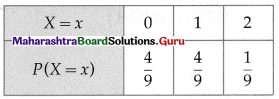Question 6.
From a lot of 30 bulbs which include 6 defectives, a sample of 4 bulbs is drawn at random with replacement. Find the probability distribution of the number of defective bulbs.
Solution:
Here, the number of defective bulbs is the random variable.
Let the number of defective bulbs be denoted by X.
∴ X can take the value 0, 1, 2, 3, 4.
Since the draws are done with replacement, therefore the four draws are independent experiments.
Total number of bulbs is 30 which include 6 defectives.
∴ P(X = 0) = P(0) = P(all 4 non-defective bulbs)
= $$\frac{24}{30} \times \frac{24}{30} \times \frac{24}{30} \times \frac{24}{30}$$
= $$\frac{256}{625}$$
P(X = 1) = P (1) = P (1 defective and 3 non-defective bulbs)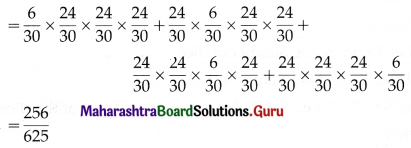P(X = 2) = P(2) = P(2 defective and 2 non-defective)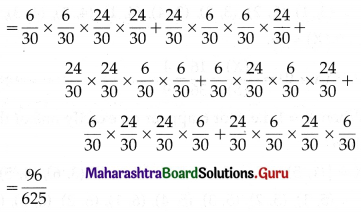P(X = 3) = P(3) = P(3 defectives and 1 non-defective)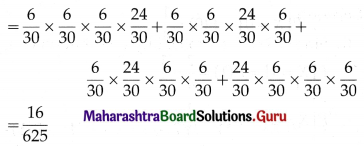P(X = 4) = P(4) = P(all 4 defectives)
= $$\frac{6}{30} \times \frac{6}{30} \times \frac{6}{30} \times \frac{6}{30}$$
= $$\frac{1}{625}$$
∴ the required probability distribution is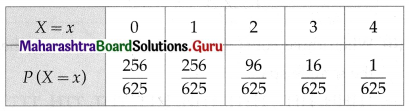Question 7.
A coin is biased so that the head is 3 times as likely to occur as the tail. If the coin is tossed twice. Find the probability distribution of a number of tails.
Solution:
Given a biased coin such that heads is 3 times as likely as tails.
∴ P(H) = $$\frac{3}{4}$$ and P(T) = $$\frac{1}{4}$$
The coin is tossed twice.
Let X can be the random variable for the number of tails.
Then X can take the value 0, 1, 2.
∴ P(X = 0) = P(HH) = $$\frac{3}{4} \times \frac{3}{4}=\frac{9}{16}$$
P(X = 1) = P(HT, TH) = $$\frac{3}{4} \times \frac{1}{4}+\frac{1}{4} \times \frac{3}{4}=\frac{6}{16}=\frac{3}{8}$$
P(X = 2) = P(TT) = $$\frac{1}{4} \times \frac{1}{4}=\frac{1}{16}$$
∴ the required probability distribution is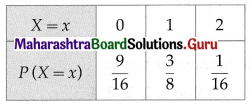Question 8.
A random variable X has the following probability distribution: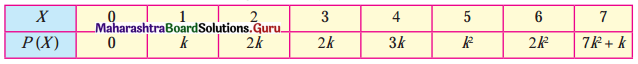Determine:
(i) k
(ii) P(X < 3) (iii) P(X > 4)
Solution:
(i) Since P (x) is a probability distribution of x,
$$\sum_{x=0}^{7} P(x)=1$$
⇒ P(0) + P(1) + P(2) + P(3) + P(4) + P(5) + P(6) + P(7) = 1
⇒ 0 + k + 2k + 2k + 3k + k2 + 2k2 + 7k2 + k = 1
⇒ 10k2 + 9k – 1 = 0
⇒ 10k2 + 10k – k – 1 = 0
⇒ 10k(k + 1) – 1(k + 1) = 0
⇒ (k + 1)(10k – 1) = 0
⇒ 10k – 1 = 0 ……..[∵ k ≠ -1]
⇒ k = $$\frac{1}{10}$$

(ii) P(X< 3) = P(0) + P(1) + P(2)
= 0 + k + 2k
= 3k
= 3($$\frac{1}{10}$$)
= $$\frac{3}{10}$$

(iii) P(0 < X < 3) = P (1) + P (2)
= k + 2k
= 3k
= 3($$\frac{1}{10}$$)
= $$\frac{3}{10}$$Question 9.
Find expected value and variance of X for the following p.m.f.: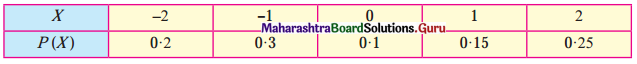Solution:
We construct the following table to calculate E(X) and V(X):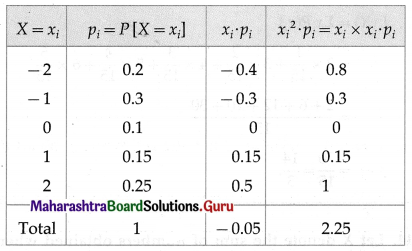From the table,
Σxipi = -0.05 and $$\Sigma x_{i}^{2} \cdot p_{i}$$ = 2.25
∴ E(X) = Σxipi = -0.05
and V(X) = $$\Sigma x_{i}^{2}+p_{i}-\left(\sum x_{i}+p_{i}\right)^{2}$$
= 2.25 – (-0.05)2
= 2.25 – 0.0025
= 2.2475
Hence, E(X) = -0.05 and V(X) = 2.2475.

Question 10.
Find expected value and variance of X, where X is the number obtained on the uppermost face when a fair die is thrown.
Solution:
If a die is tossed, then the sample space for the random variable X is
S = {1, 2, 3, 4, 5, 6}
∴ P(X) = $$\frac{1}{6}$$; X = 1, 2, 3, 4, 5, 6.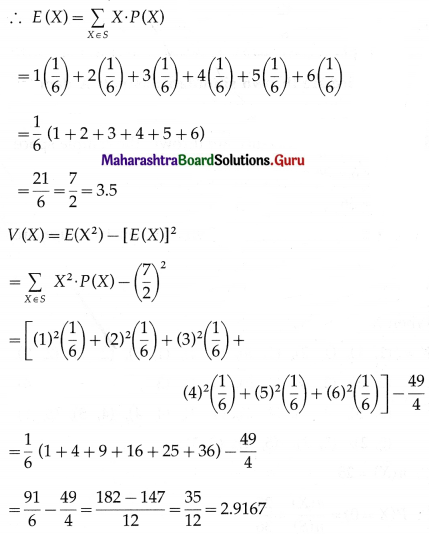Hence, E(X) = 3.5 and V(X) = 2.9167.

Question 11.
Find the mean number of heads in three tosses of a fair coin.
Solution:
When three coins are tossed the sample space is {HHH, HHT, THH, HTH, HTT, THT, TTH, TTT}
∴ n(S) = 8
Let X denote the number of heads when three coins are tossed.
Then X can take values 0, 1, 2, 3
P(X = 0) = P(0) = $$\frac{1}{8}$$
P(X = 1) = P(1) = $$\frac{3}{8}$$
P(X = 2) = P(2) = $$\frac{3}{8}$$
P(X = 3) = P(3) = $$\frac{1}{8}$$
∴ mean = E(X) = ΣxiP(xi)
= $$0 \times \frac{1}{8}+1 \times \frac{3}{8}+2 \times \frac{3}{8}+3 \times \frac{1}{8}$$
= $$0+\frac{3}{8}+\frac{6}{8}+\frac{3}{8}$$
= $$\frac{12}{8}$$
= 1.5Question 12.
Two dice are thrown simultaneously. If X denotes the number of sixes, find the expectation of X.
Solution:
When two dice are thrown, the sample space S has 6 × 6 = 36 sample points.
∴ n(S) = 36
Let X denote the number of sixes when two dice are thrown.
Then X can take values 0, 1, 2
When X = 0, then
X = {(1, 1), (1, 2), (1, 3), (1, 4), (1, 5), (2, 1), (2, 2), (2, 3), (2, 4), (2, 5), (3, 1), (3, 2), (3, 3), (3, 4), (3, 5), (4, 1), (4, 2), (4, 3), (4, 4), (4, 5), (5, 1), (5, 2), (5, 3), (5, 4), (5, 5)}
∴ n(X) = 25
∴ P(X = 0) = $$\frac{n(X)}{n(S)}=\frac{25}{36}$$
When X = 1, then
X = {(1, 6), (2, 6), (3, 6), (4, 6), (5, 6), (6, 1), (6, 2), (6, 3), (6, 4), (6, 5)}
∴ n(X) = 10
∴ P(X = 1) = $$\frac{n(X)}{n(S)}=\frac{10}{36}$$
When X = 2, then X = {(6, 6)}
∴ n(X) = 1
∴ P(X = 2) = $$\frac{n(X)}{n(S)}=\frac{1}{36}$$
∴ E(X) = ΣxiP(xi)
= $$0 \times \frac{25}{36}+1 \times \frac{10}{36}+2 \times \frac{1}{36}$$
= $$0+\frac{10}{36}+\frac{2}{36}$$
= $$\frac{1}{3}$$

Question 13.
Two numbers are selected at random (without replacement) from the first six positive integers. Let X denote the larger of the two numbers. Find E(X).
Solution:
Two numbers are chosen from the first 6 positive integers.
∴ n(S) = $${ }^{6} C_{2}=\frac{6 \times 5}{1 \times 2}$$ = 15
Let X denote the larger of the two numbers.
Then X can take values 2, 3, 4, 5, 6.
When X = 2, the other positive number which is less than 2 is 1.
∴ n(X) = 1
∴ P(X = 2) = P(2) = $$\frac{n(X)}{n(S)}=\frac{1}{15}$$
When X = 3, the other positive number less than 3 can be 1 or 2 and hence can be chosen in 2 ways.
∴ n(X) = 2
P(X = 3) = P(3) = $$\frac{n(X)}{n(S)}=\frac{2}{15}$$
Similarly, P(X = 4) = P(4) = $$\frac{3}{15}$$
P(X = 5) = P(5) = $$\frac{4}{15}$$
P(X = 6) = P(6) = $$\frac{5}{15}$$
∴ E(X) = ΣxiP(xi)
= $$2 \times \frac{1}{15}+3 \times \frac{2}{15}+4 \times \frac{3}{15}+5 \times \frac{4}{15}+6 \times \frac{5}{15}$$
= $$\frac{2+6+12+20+30}{15}$$
= $$\frac{70}{15}$$
= $$\frac{14}{3}$$Question 14.
Let X denote the sum of numbers obtained when two fair dice are rolled. Find the standard deviation of X.
Solution:
If two fair dice are rolled then the sample space S of this experiment is
S = {(1, 1), (1, 2), (1, 3), (1, 4), (1, 5), (1, 6), (2, 1), (2, 2), (2, 3), (2, 4), (2, 5), (2, 6), (3, 1), (3, 2), (3, 3), (3, 4), (3, 5), (3, 6), (4, 1), (4, 2), (4, 3), (4, 4), (4, 5), (4, 6), (5, 1), (5, 2), (5, 3), (5, 4), (5, 5), (5, 6), (6, 1), (6, 2), (6, 3), (6, 4), (6, 5), (6, 6)}
∴ n(S) = 36
Let X denote the sum of the numbers on uppermost faces.
Then X can take the values 2, 3, 4, 5, 6, 7, 8, 9, 10, 11, 12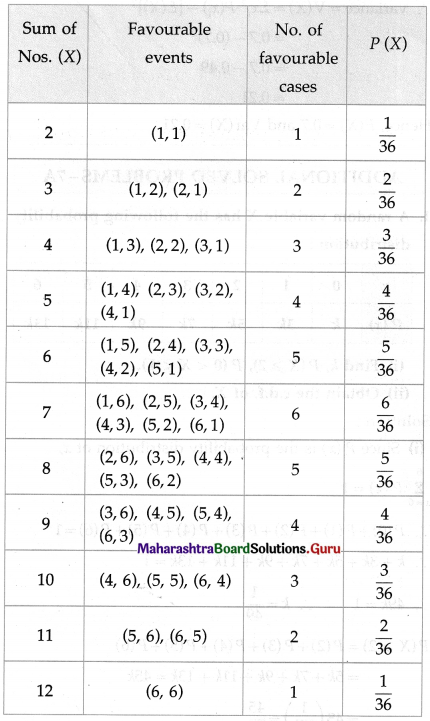∴ the probability distribution of X is given by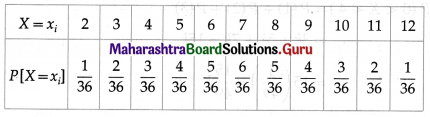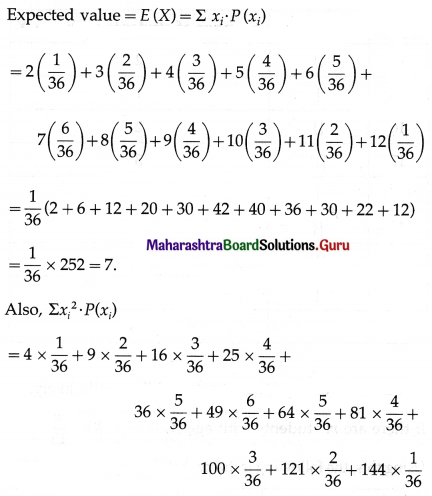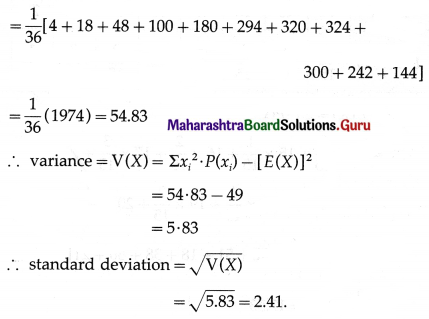Question 15.
A class has 15 students whose ages are 14, 17, 15, 14, 21, 17, 19, 20, 16, 18, 20, 17, 16, 19 and 20 years. One student is selected in such a manner that each has the same chance of being chosen and the age X of the student is recorded. What is the probability distribution of the random variable X? Find mean, variance, and standard deviation of X.
Solution:
Let X denote the age of the chosen student. Then X can take values 14, 15, 16, 17, 18, 19, 20, 21.
We make a frequency table to find the number of students with age X: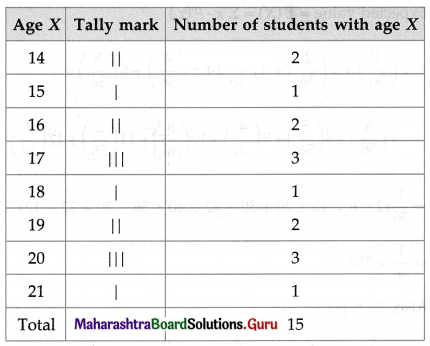The chances of any student selected are equally likely.
If there are m students with age X, then P(X) = $$\frac{m}{15}$$
Using this, the following is the probability distribution of X: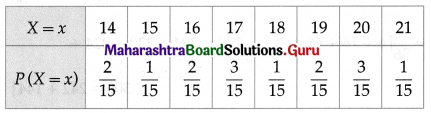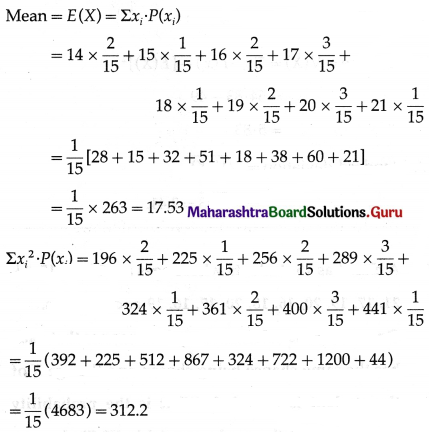Variance = V(X) = $$\Sigma x_{i}^{2}$$ . P(xi) – [E(X)]2
= 312.2 – (17.53)2
= 312.2 – 307.3
= 4.9
Standard deviation = √V(X) = √4.9 = 2.21
Hence, mean = 17.53, variance = 4.9 and standard deviation = 2.21.Question 16.
In a meeting, 70% of the member’s favour and 30% oppose a certain proposal. A member is selected at random and we take X = 0 if he opposed and X = 1 if he is in favour. Find E(X) and Var(X).
Solution:
X takes values 0 and 1.
It is given that
P(X = 0) = P(0) = 30% = $$\frac{30}{100}$$ = 0.3
P(X = 1) = P(1) = 70% = $$\frac{70}{100}$$ = 0.7
∴ E(X) = Σxi . P(xi) = 0 × 0.3 + 1 × 0.7 = 0.7
Also, $$\Sigma x_{i}^{2} \cdot P\left(x_{i}\right)$$ = 0 × 0.3 + 1 × 0.7 = 0.7
∴ Variance = V(X) = $$\Sigma x_{i}^{2} \cdot P\left(x_{i}\right)-[E(X)]^{2}$$
= 0.7 – (0.7)2
= 0.7 – 0.49
= 0.21
Hence, E(X) = 0.7 and Var(X) = 0.21.